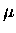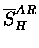Next: Corrections to in the Up: Electron telescope measurements Previous: Electron telescope measurements

### Energy dependence of the stopping fraction

We plot in the top of Fig. 6.6 the telescope energy spectra, e.g., the neutron detector dynode output, recorded in coincidence with the corresponding scintillator pair. The time cut of t>0.02s was applied to remove the prompt beam signal (which would otherwise slightly increase counts at energies less than 200 ch). With the minimum ionizing energy loss MeV/(g/cm2) for scintillator materials, and our detector depth being about 10 cm (4 inches), the minimum ionizing electrons deposit energy of order 20 MeV. The muon decay electrons (in free space) range from 0 to about 53 MeV in energy, hence some would stop in the detector, while others would go through. Thus, the peak in Fig. 6.6 (above) near channel 700 should be due to the minimum ionizing electrons corresponding to some 20 MeV. Detailed GEANT calculations indeed confirm this picture .

The bottom figure in Fig. 6.6 illustrates the reduced muon stopping fraction in hydrogen determined from the amplitude ratio of a two-exponential lifetime fit,( =A2/(A1/Q1 + A2)), as a function of the energy deposit in the NE213 detector. The fits were performed with the various energy cuts, with their intervals indicated roughly by the position and width of the histogram bars. This clearly demonstrates that the stopping fraction determined from the amplitude ratio is dependent on the energies to which the detector is sensitive. Possible mechanisms for this energy dependence are considered in the following sections, and will lead to the corrections to.Next: Corrections to in the Up: Electron telescope measurements Previous: Electron telescope measurements